# Rubik's Cube Nuggets

### Solving the cube using computer-generated sequences of moves - by Don Cross

This web page contains useful tricks for solving Rubik's Cube that were found by a computer program I wrote in June 2002. The computer program simulates a Rubik's Cube and tries millions of different combinations of moves, until it finds combinations that affect the corners and edges on only one of the 6 planes of the cube. The original document was a Microsoft Excel spreadsheet. I optically scanned the diagrams from an old printout of that spreadsheet, hence the somewhat degraded image quality.

## Glossary

Note that many of the words are defined in terms of each other. When a defined word appears in the definition of another word, the defined word is italicized.

 word definition corner One of the 8 pieces of the Rubik's Cube that has 3 tiles on it. cost The number of face rotations needed to perform a nugget. cycle To move 3 edges/corners from position A to position B, B to C, and C to A. edge One of the 12 pieces of the Rubik's Cube that has 2 tiles on it. flip To rotate the orientation of an edge (there are 2 possible orientations). mirror The mirror image of a nugget, with the mirror held parallel to the cube's right face. nugget A sequence of moves on the Rubik's Cube that performs a desired operation. reverse The inverse of a nugget, meaning the sequence of moves that would undo the nugget. spin To rotate the orientation of a corner (there are 3 possible orientations). swap To move 2 edges/corners from position A to B and B to A. tile One of the colored faces of either an edge or a corner.

## Move Notation

 `f` front `p` posterior `r` right `l` left `t` top `b` bottom `x` right and left (`x+` = `r+l-`) `y` front and posterior (`y-` = `f-p+`) `z` top and bottom (`z+` = `t+b-`)
 `+` clockwise (looking into the moved face) `-` anticlockwise (looking into the moved face) `2` 180-degree turn

 N1: Cycles 3 corners on the top face, spinning one clockwise and one anticlockwise. ``` f+ r+ f- l+ f+ r- f- l- ```N2: Cycles 3 edges without flipping any of them. ``` f2 t+ x- f2 x+ t+ f2 ```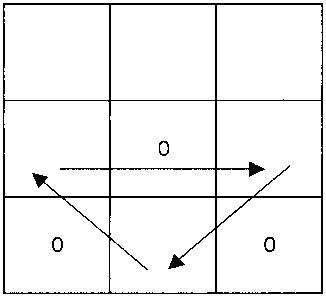N3: Cycles 3 edges, flipping 2. ``` x- f+ x+ t2 x- f+ x+ ```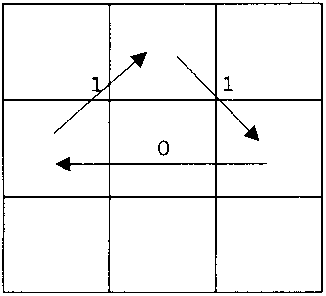N4: Swaps 2 corners and 2 edges, with no flipping/spinning. ``` f2 r+ t+ r- f2 l+ b- l+ b+ l2 ```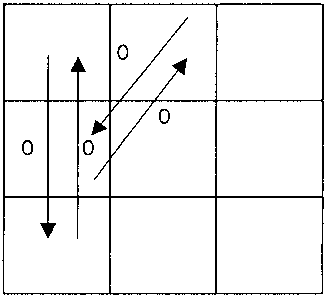N5: Spins 3 corner cubes anticlockwise and cycles 3 edge cubes. ``` r- t- r+ t- r- t2 r+ t2 ```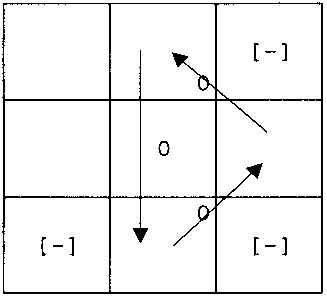N6: Cycles 3 edges, flipping 2 of them. ``` f- t- f+ x- b- f+ b+ x+ ```N7: Swaps 2 pairs of opposite edges, flipping one in each pair. ``` f- t- l- t2 l+ f+ t+ r+ t2 r- ```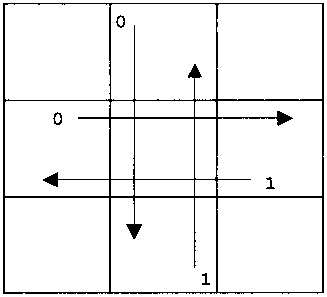N8: Spins 3 corners anticlockwise, and flips 2 edges. ``` r+ t+ r2 f+ r+ f2 t+ f+ t2 ```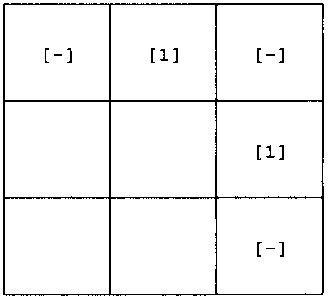N9: Cycles 3 corners, spinning each clockwise. ``` f+ r- t2 r+ f- r- f+ t2 f- r+ ```N10: Swaps both diagonally opposite corners, with no spinning of any. ``` f2 x2 p2 b- f2 x2 p2 t- ```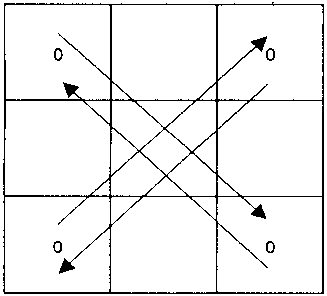N11: Flips 2 adjacent edges. ``` t+ f+ t+ f2 b- l- t- l+ t+ b+ f2 t2 f- ```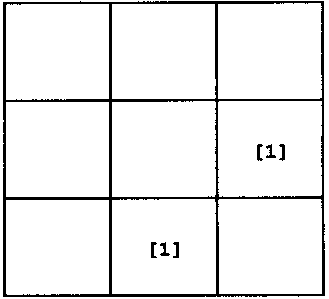N12: Flips 2 opposite edges. ``` t+ y+ r- t+ f- r+ t- y- l+ t- f+ l- ```N13: Spins 2 opposite corners. ``` t2 r+ f- b2 f+ r- t2 r+ f- b2 f+ r- ```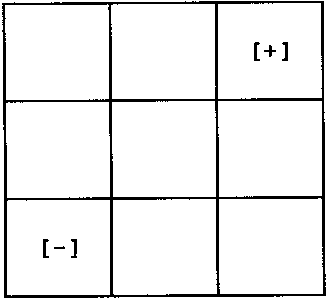N14: Flips all 4 edges on top face. ``` t+ x+ f2 r2 b2 r2 p+ r2 b2 r2 f2 x- ```